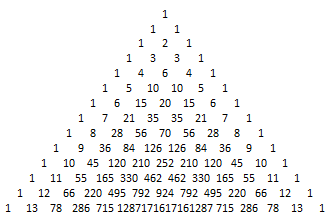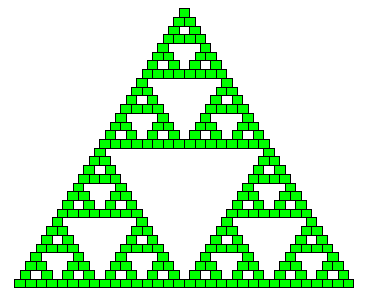# Properties of Pascal Triangle

Warning! Some information on this page is older than 5 years now. I keep it for reference, but it probably doesn't reflect my current knowledge and beliefs.

Thu
12
Nov 2009

Pascal Triangle is a mathematical object that looks like triangle with numbers arranged the way like bricks in the wall. Each next row has one more number, ones on both sides and every inner number is the sum of two numbers above it. It can span infinitely.Despite simple algorithm this triangle has some interesting properties. First, if you draw only odd numbers, you get a fractal - Sierpinski Triangle.Second, graph of numbers in each row resembles Gaussian distribution function. The lower row you take from the triangle (containing more numbers), the more precise graph you get.I've made these images with Octave - a free Matlab alternative. I like it and I think concepts behind this Matlab programming language can be quite useful for doing computations, but doing graphics is painfully slow. Whole seconds to draw several dozens of texts or rectangles? WTF?!